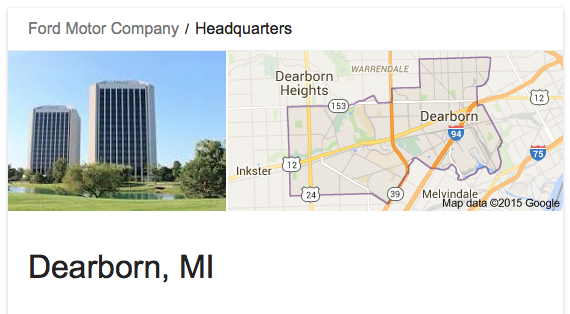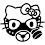## Thursday, December 24, 2015

### 33 47 74 113 137 | Priscilla Ford, the Woman Who Ran Over Reno Pedestrians on Thanksgiving Day, November 27, 19807 Dead, 22 Injured, where have we seen these numbers? (22 divided by 7, is Pi) (Lincoln died at 7:22) Ford, from Michigan???
https://en.wikipedia.org/wiki/Priscilla_Ford

In light of the December 20, 2015, Las Vegas, 'mad motorists incident', where supposedly people walking on the sidewalk were mowed down by an angry mother behind the wheel, it reminds of a story from Reno, Nevada, 1980, 35-years earlier.

The accused in the 1980 story, was 'Priscilla Joyce Ford'.

Priscilla = 7+9+9+1+3+9+3+3+1 = 45/54 (Dearborn, MI = 54)
Joyce = 1+6+7+3+5 = 22
Ford = 6+6+9+4 = 25
Priscilla Joyce Ford = 92/101 (Assassin = 101)The "mad motorist Ford" from Michigan...

Priscilla = 16+18+9+19+3+9+12+12+1 = 99
Joyce = 10+15+25+3+5 = 58 (Freemasonry)
Ford = 6+15+18+4 = 43 (The name of a motor company) (Massacre)
Priscilla Joyce Ford = 200

She was born February 10, 1929.

2/10/1929 = 2+10+19+29 = 60
2/10/1929 = 2+10+(1+9+2+9) = 33
2/10/1929 = 2+1+0+1+9+2+9 = 24
2/10/29 = 2+10+29 = 41 (February 10, is the 41st day of the year)

Priscilla would die in a women's prison, in Nevada, at age 75, just shy of 76-years old.

1/29/2005 = 1+29+20+05 = 55
1/29/2005 = 1+29+(2+0+0+5) = 37
1/29/2005 = 1+2+9+2+0+0+5 = 19
1/29/05 = 1+29+05 = 35

With regards to the number 35, the Nevada 'car terror incidents from black women' come 35-years apart.Lincoln declared the first annual Thanksgiving Holiday, 74-years after George Washington celebrated the first ThanksgivingHoliday = 8+15+12+9+4+1+25 = 74
Notice the date of the incident, Thanksgiving Day, November 27, 1980.

11/27/1980 = 11+27+19+80 = 137 (33rd Prime)
11/27/1980 = 11+27+(1+9+8+0) = 56
11/27/1980 = 1+1+2+7+1+9+8+0 = 29
11/27/80 = 11+27+80 = 118 (Death, Jewish Gematria)

Aside from the familiar date numerology, what else stands out about this incident, is the year of the car, 1974, emphasis on '74.  In the more recent incident, they said the car was a 1996, emphasis on '96, Oldsmobile.

Masonic = 13+1+19+15+14+9+3 = 74
Freemason = 6+18+5+5+13+1+19+15+14 = 96

The car was also a 'Lincoln'.

Of course the Freemasons have an obsession with the number 33, a number coded into the date of the incident and the birth of the driver.

From 'news review', here is an account of what happened the day Ford ran over dozens in Reno, NV, and what happened in the trial that followed.  As you read, notice the purposefully encoded Masonic Numbers.  Also notice that Ford is from Michigan, like the auto manufacturer...

https://www.newsreview.com/reno/day-terror-came-downtown/content?oid=20584

At around 47 degrees?  Are they serious?  Yes, sadly, they are.

Recall, the recent 'car terror in Nevada' incident came on a date with '47' numerology.

All of the stories mentioned, are stories by the numbers.  The Son of Sam and John Hinckley, are most definitely hoaxes.

Again, the name 'Ford', in light of a 'motor tragedy'.

Notice the reporting.  The 59th minute, when the word 'kill' has a jewish gematria of 59.  And then adding insult to injury, the car travels another '322 feet' down the street?  Oh yea?  Who got that measurement?

The description of this incident, sounds like the initials description of the Boston Marathon Bombing.  That said, a man has already written me, saying he worked in Reno at the time of this incident, and saying the carnage was very real.  Take that for whatever it's worth.

Former teacher, Priscilla Ford?  Let us not forget, that in numerology, '33' is the 'master teacher' number.

Teacher = 2+5+1+3+8+5+9 = 33

John Oakes, the right man to be commenting on a scene of death, in Nevada.

John = 1+6+8+5 = 20
Oakes = 6+1+2+5+1 = 15/24
John Oakes = 35/44

John = 10+15+8+14 = 47 (Nevada)
Oakes = 15+1+11+5+19 = 51
John Oakes = 98

With regards to Thanksgiving, it reminds of how the more recent incident, December 20, 2015, took place with a '96 Oldsmobile.

Thanksgiving = 2+8+1+5+2+1+7+9+4+9+5+7 = 60/69/78/96

It sounds like she would have been a good fit for the Pentagon.  This seems to be the same care the employees of that building take to human life.

Notice the trial began January 29, before it being ordered that Ford receive mental treatment, beginning the 119th day of the year.  Then on August 4, the 216th day of the year, Obama's birthday, she was found competent for trial.

1/29/1981 = 1+29+19+81 = 130
1/29/1981 = 1+29+(1+9+8+1) = 49
1/29/1981 = 1+2+9+1+9+8+1 = 31
1/29/81 = 1+29+81 = 111

4/29/1981 = 4+29+19+81 = 133
4/29/1981 = 4+29+(1+9+8+1) = 52
4/29/1981 = 4+2+9+1+9+8+1 = 34
4/29/81 = 4+29+81 = 114

8/4/1981 = 8+4+19+81 = 112
8/4/1981 = 8+4+1+9+8+1 = 31
8/4/81 = 8+4+81 = 93

11/12/1981 = 11+12+19+81 = 123 (Conspiracy)
11/12/1981 = 11+12+(1+9+8+1) = 42
11/12/1981 = 1+1+1+2+1+9+8+1 = 24
11/12/81 = 11+12+81 = 104

Interesting testimony.

Notice the date of the death verdict, 33-years ago.

3/28/1982 = 3+28+19+8 = 3+28+19+82 = 132
3/28/1982 = 3+28+(1+9+8+2) = 51
3/28/1982 = 3+2+8+1+9+8+2 = 33
3/28/82 = 3+28+82 = 113

With regards to the '74 Lincoln and Thanksgiving, let's not forget the following.

This November 26 will make 74-years since FDR declared Thanksgiving to be celebrated on the fourth Thursday of each November.  He made that declaration on November 26, 1941.  Lincoln also declared November 26, 1863 to be the beginning of an annual Thanksgiving Celebration, 74-years after George Washington had the first national Thanksgiving Day.  In other words, November 26 and Thanksgiving have been big in history.

Holiday = 8+15+12+9+4+1+25 = 74

1. First Thanksgiving November 26, 1789 (Washington)
2. Thanksgiving made annual holiday November 26, 1863 (Lincoln)
3. Thanksgiving made fourth Thursday of every November, November 26, 1941 (FDR)
Here is also a quick little blurb about the original Christian Pilgrims, who celebrated the First Thanksgiving.  Notice the date they left, September 6, and the number of them, 102.

Freemason = 96
Thanksgiving = 96 (Pythagorean, with SKV exceptions)
United States of America = 102 (Pythagorean, S exceptions)

The first ever Thanksgiving, long before it was a holiday, was celebrated December 13, 1621.

1.What jumped out to me here is the 7 dead 22 wounded, like the approximation of pi. Like Lincoln pronounced dead at 7:22 and she's driving a Lincoln. Like Reagan fake shot at 2:27 - an incident that occurred exactly 123 days after this incident of MK-induced road rage (or 4 months 3 days and Ford =43).

1.And notice she's throwing up the pyramid.

2.Yea, that definitely stands out. I meant to comment on that, good eyes.

3.I also forgot to emphasize that 'Ford' was from 'Michigan', home of the 'Motor City'.

2.Great post! I have to mull this over, but they do love Pi code and Thanksgiving is a great target for it.
schizophrenia 151(EO)

3.Main dessert for thanksgiving, Pi?